Courses

# Availability, Irreversibility, Thermodynamic Relations - MCQ Test 2

## 30 Questions MCQ Test Mock Test Series - Mechanical Engineering (ME) for GATE 2020 | Availability, Irreversibility, Thermodynamic Relations - MCQ Test 2

Description
This mock test of Availability, Irreversibility, Thermodynamic Relations - MCQ Test 2 for Mechanical Engineering helps you for every Mechanical Engineering entrance exam. This contains 30 Multiple Choice Questions for Mechanical Engineering Availability, Irreversibility, Thermodynamic Relations - MCQ Test 2 (mcq) to study with solutions a complete question bank. The solved questions answers in this Availability, Irreversibility, Thermodynamic Relations - MCQ Test 2 quiz give you a good mix of easy questions and tough questions. Mechanical Engineering students definitely take this Availability, Irreversibility, Thermodynamic Relations - MCQ Test 2 exercise for a better result in the exam. You can find other Availability, Irreversibility, Thermodynamic Relations - MCQ Test 2 extra questions, long questions & short questions for Mechanical Engineering on EduRev as well by searching above.
QUESTION: 1

### For a reversible power cycle, the operating temperature limits are 800 K and 300 K. It takes 400 kJ of heat. The unavailable work will be:

Solution:

Available part of the heat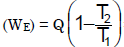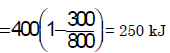Unavailable work (Wu) = 400 – 250 = 150 kJ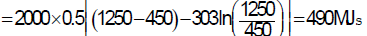QUESTION: 2

Solution:
QUESTION: 3

### The loss due to irreversibility in the expansion valve of a refrigeration cycle shown in the given figure is represented by the area under the line.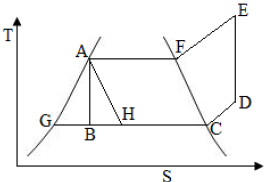Solution:

Entropy will increase in the process AH is BH.

Therefore Irreversibility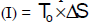i.e. area under the line BH.

QUESTION: 4

Assertion (A): The first-law efficiency evaluates the energy quantity utilization, whereas the second-law efficiency evaluates the energy quality utilization.
Reason (R):  The second-law efficiency for a process is defined as the ratio of change of available energy of the source to the change of available energy of the system.

Solution: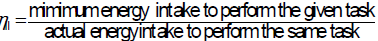QUESTION: 5

Considering the relationship TdS = dU + pdV between the entropy (S), internal energy (U), pressure (p), temperature (T) and volume (V), which of the following statements is correct?

Solution:
QUESTION: 6

The specific heats of an ideal gas depends on its

Solution:
QUESTION: 7

A 2 kW, 40 litre water heater is switched on for 20 minutes. The h eat capacity Cp for water is 4.2 kJ/kg K. Assuming all the electrical energy has gone into heating the water, increase of the water temperature in degree centigrade is:

Solution:

Heat absorbed by water = Heat supplied by heater.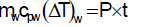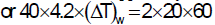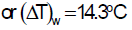QUESTION: 8

A positive value to Joule-Thomson coefficient of a fluid means

Solution: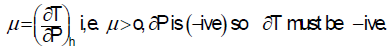QUESTION: 9

Match 4 correct pairs between List-I and List-II for the questions [GATE-1994] For a perfect gas: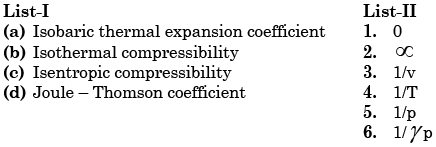Solution:
QUESTION: 10

Which thermodynamic property is evaluated with the help of Maxwell equations from the data of other measurable properties of a system?

Solution:

From Maxwell relation Clapeyron equation comes.

QUESTION: 11

Clapeyron‟s equation is used for finding out the

Solution:
QUESTION: 12

Which one of the following statements applicable to a perfect gas will also be true for an irreversible process? (Symbols have the usual meanings).

Solution:

The relations in (a) and (b) are applicable for a reversible processes and (c) Tds = du + pdV is a relation among properties which are independent of the path.

QUESTION: 13

Assertion (A): Specific heat at constant pressure for an ideal gas is always greater than the specific heat at constant volume.

Reason (R): Heat added at constant volume is not utilized for doing any external work.

Solution:
QUESTION: 14

As compared to air standard cycle, in actual working, the effect of variations in specific heats is to:

Solution:
QUESTION: 15

Molal specific heats of an ideal gas depend on

Solution:

For ideal gas CP and CV are constant but mole is depends on the number of atoms in a molecule.

QUESTION: 16

The difference between constant pressure specific heat Cp and constant volume specific heat Cv for pure substance

Solution:
QUESTION: 17

Joule-Thomson coefficient is the slope of

Solution:
QUESTION: 18

Which gas shows a heating effect in the Joule-Thomson experiment, while undergoing throttling process through a porous plug of cotton wool?

Solution:
QUESTION: 19

Joule – Thomson coefficient is the ratio of

Solution:

Joule Thomson coefficient is the ratio of temperature change to pressure change when a gas undergoes adiabatic throttling.

QUESTION: 20

Consider the following statements in respect of the Clausius – Clapeyron equation:

1. It points to one possible way of measuring thermodynamic temperature.

2. It permits latent heat of vaporization to be estimated from measurements of specific volumes of saturated liquid, saturated vapour and the saturation temperatures at two nearby pressures.

3. It does not apply to changes from solid to the liquid phase and from solid to the Vapour phase.

Which of the statements given above are correct?

Solution:
QUESTION: 21

The variation of saturation pressure with saturation temperature for a liquid is 0.1 bar/K at 400 K. The specific volume of saturated liquid and dry saturated vapour at 400 K are 0.251 and 0.001 m3/kg What will be the value of latent heat of vaporization using Clausius Clapeyron equation?

Solution: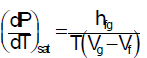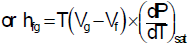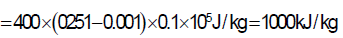QUESTION: 22

Clausius-Clapeyron equation gives the 'slope' of a curve in

Solution:
QUESTION: 23

Gibb's phase rule is given by:

(F = number of degrees of freedom; C = number of components; P = number of phases)

Solution:

F = C – P + 2

QUESTION: 24

Which one of the following relationships defines the Helmholtz function F?

Solution:
QUESTION: 25

Consider the following statements:

1. Azeotropes are the mixtures of refrigerants and behave like pure substances. 2

. Isomers refrigerants are compounds with the same chemical formula but have different molecular structures.

3. The formula n + p + q = 2m is used for unsaturated chlorofluorocarbon compounds (m, n, p and q are the numbers atoms of carbon, hydrogen, fluorine and chlorine respectively).

Which of these statements are correct?

Solution:

Isomers: Compounds with the same chemical formula but different molecular structure.

QUESTION: 26

Which one of the following expressions for T ds is true for a simple compressible substance? (Notations have the usual meaning)

Solution:

dQ = dh – Vdp or Tds = dh – Vdp

QUESTION: 27

Assertion (A): Specific heat at constant pressure for an ideal gas is always greater than the specific heat at constant volume.

Reason (R): Heat added at constant volume is not utilized for doing any external work.

Solution:

Both A and R correct and R is the correct explanation of A

QUESTION: 28

Which one of the following properties remains unchanged for a real gas during Joule-Thomson process?

Solution:
QUESTION: 29

Assertion (A): Water will freeze at a higher temperature if the pressure is increased.

Reason (R): Water expands on freezing which by Clapeyron's equation gives negative slope for the melting curve.

Solution:
QUESTION: 30

Which one of the following relationships defines Gibb's free energy G?

Solution: